# Crushing Test of Stones | Testing of Stones | Stone As A Building Material | Stones | Construction Materials | By Ashutosh Nautiyal

1. Compressive Strength Test (IS: 1121 (Part I) 2. Transverse Strength Test (IS: 1121 (Part II) 3. Tensile Strength Test (IS: 1121 (Part III))

## Crushing Test On Stones

### Requirements:

• Take samples of stones weighing at least 25 kg each.

• Specimen species should be in the form of cubes or cylinders.

• The lateral dimension or diameter should be of 50 mm at least.

• The ratio of lateral dimension or ratio of height to diameter should be 1:1.

• A minimum of three specimens should be used for testing.

#### Procedure:

• Specimen is immersed in water at 20 to 30 °C for 72 hours. (Test in saturated condition)

• Specimen is dried in an oven at 105 ± 5 °C for 24 hours. (Test in dry condition)

• Then it is cooled in a desiccator to 20 to 30 °C.

• Later, it is tested in Universal Testing Machine.

• The load is gradually applied at a rate of 14 N /sq. mm /min (14 newtons per millimetre square per minute) until the specimen breaks down.
The Compressive Strength is then calculated by the formula:

*When the ratio of lateral dimension or ratio of height to diameter differs from unity by 25% or more then Compressive strength in Calculated by this formula:

here,

Cp= compressive strength of standard specimen piece

b= diameter or lateral dimension

h= height

### Revision Mind Map For Compressive Strength Test on Stone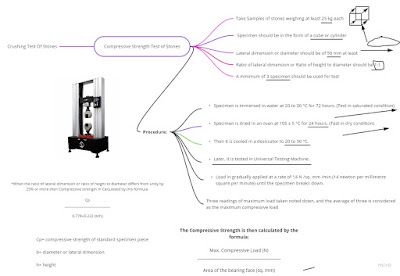Revision Mind Map For Compressive Strength Test on Stone

### 2.  Transverse Strength Test (IS: 1121 (Part II)

#### Requirements:-

• Test pieces should be blocks of the size of 200 mm * 50 mm * 5 mm.

• A suitable form of apparatus is required to perform the tensile strength test on stones.

#### Procedure:-

• These test pieces are used to determine the transverse strength of the stone in each saturated(immersed in water at 20 to 30°C for 3 days) and dry condition(dried in an oven at 105±5°C for 24 hours and cooled in a desiccator to 20 to 30°C).

• Each specimen piece is supported upon two self-aligning bearers A and B(40 mm in diameter each) and the distance between centres of bearers being 150 mm.

• Bearer A is supported horizontally on two bearer screws C, which carry hardened steel balls D.

• Bearer B is supported on one such screw and ball.Image From Book By S.K. Duggal

• The load is then applied centrally on the specimen piece at a uniform rate of 2 kN/min through a bearer E(40 m in diameter) placed midway between the supports upon the upper surface of the specimen S and parallel to the supports.

• The Transverse Strength(R) of the specimen is given by:-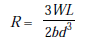Transverse Strength

where R=Transverse Strength in N/mm2

W= Central breaking load in N

L=Length Of Span in mm

b= Average Width in mm of the test piece at the midsection

d=Average Depth in mm of the test piece at the midsection

• The average of three results(separately for saturated and dry condition) should be taken to determine the transverse strength of the stone.

#### Precaution:-

• If any test piece is giving result as much as 15% below the average value, it should be examined for defects.

### 3.  Tensile Strength Test (IS: 1121 (Part III))

#### Requirements:-

• A testing machine of sufficient capacity for the tests and capable of applying load at the specified rate should be used.

• Take three cylindrical test pieces of diameter greater than or equal to 50 mm.

•  The ratio of diameter to height should be 1:2 for the test pieces.

• The testing machine should be equipped with two steel plates of width 25 mm, thickness 10 mm and length equal to the length of the test piece.

#### Procedure:-

• These test pieces are used to determine the tensile strength of the stone in each saturated(immersed in water at 20 to 30°C for 3 days) and dry condition(dried in an oven at 105±5°C for 24 hours and cooled in a desiccator to 20 to 30°C room temperature).

• Each test piece to be tested is sandwiched between two steel plates of the dimensions mentioned above.

• Now, the load is applied without shock and increased continuously at a uniform rate until the specimen splits and no greater load is sustained by it.Image From Book By S.K. Duggal

• Then, the maximum load applied to the specimen is recorded.

• Finally, the Split Tensile Strength(S) of the stone under observation is calculated by using the formula specified below:-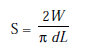Split Tensile Strength

where S=split tensile strength (N/mm2)

d=diameter of specimen(mm)

L=length of specimen(mm)

• The average of three results separately for each condition should be recorded as the split tensile strength of the sample.

#### Precaution:-

• In any case, if any test piece gives a value of as much as 15% below the average, it should be examined for defects and if found defective the test piece should be rejected.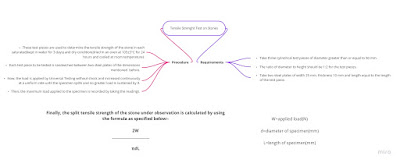Mind Map for Tensile Strenght test on stones

### 4.  Absorption Test (IS: 1124)

#### Requirements:-

• The test pieces of stone which are crushed or broken and which are passed through 20 mm IS sieve and retained on 10 mm IS Sieve.

• Apparatus such as a glass vessel, weighing balance, thermostatically controlled oven, desiccator, 1000 ml capacity measuring cylinder, 100 ml measuring cylinder, distilled water and two dry clothes must be available to perform the test.

#### Procedure:-

• The test piece weighing about 1 kg is washed to remove the dust particles.

• Then, it is immersed in distilled water in a glass vessel at room temperature(20 to 30°C)for 24 hours.

• The entrapped air is removed by gentle agitation(achieved by rapid clock-wise and anti-clockwise rotation of the vessel) soon after immersion and again at the end of the soaking period.

• The vessel is then emptied and the test piece is allowed to drain.

• The test piece is then placed on a dry cloth and the surface is dried with the cloth gently.

• When the first cloth removes no further moisture, it is transferred to a second dry cloth.

• The test piece is spread out not more than once on the second cloth and it is left exposed to the atmosphere away from sunlight or any other source of heat for not less than 10 minutes until it appears to be completely surface dry.

• The sample is then weighed (B).

• Then, the sample is carefully introduced in a 1000 ml capacity measuring cylinder and distilled water is poured by means of 100 ml capacity measuring cylinder until the level of water in the larger cylinder reaches 1000 ml mark(while taking care to remove the entrapped air).

• The quantity of water added to the sample is recorded in ml or expressed in gram weight (C).

• The water in the larger cylinder is then drained and the sample is carefully taken out and dried in an oven at 100 to 110°C for not less than 24 hours.

• It is then cooled in a desiccator to room temperature and weighed (A).

• The room temperature during the test is recorded.

• Finally, the Apparent Specific Gravity is calculated by using the formula specified below:-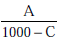Apparent Specific Gravity

where A is the weight of oven-dry test piece (g)

C is the quantity of water added in 1000 ml jar containing the test piece (g)

• The Apparent Specific Gravity shall be expressed as a numerical value for the saturated surface-dry sample at the recorded temperature and it shall be the average of three determinations.

• Similarly, Water Absorption is calculated by using the formula specified below:-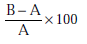Water Absorption

where A is the weight of oven-dry test piece (g)

B is the weight of saturated surface-dry test piece (g)

• The Water Absorption shall be expressed as a percentage by weight of the oven-dry sample and it shall be the average of three determinations.

•  Now, the Apparent Porosity is calculated by using the formula specified below:-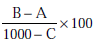Apparent Porosity

where A is the weight of oven-dry test piece (g)

B is the weight of saturated surface-dry test piece (g)

C is the quantity of water added in 1000 ml jar containing the test piece (g)

• The Apparent Porosity shall be expressed as a percentage and shall be the average of three determinations.

• Similarly, the True Porosity is calculated by using the formula specified below:-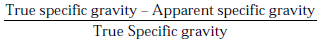True Porosity

*Self Typed

*Source- Internet, Books and Self-Analysis

Getting Info...